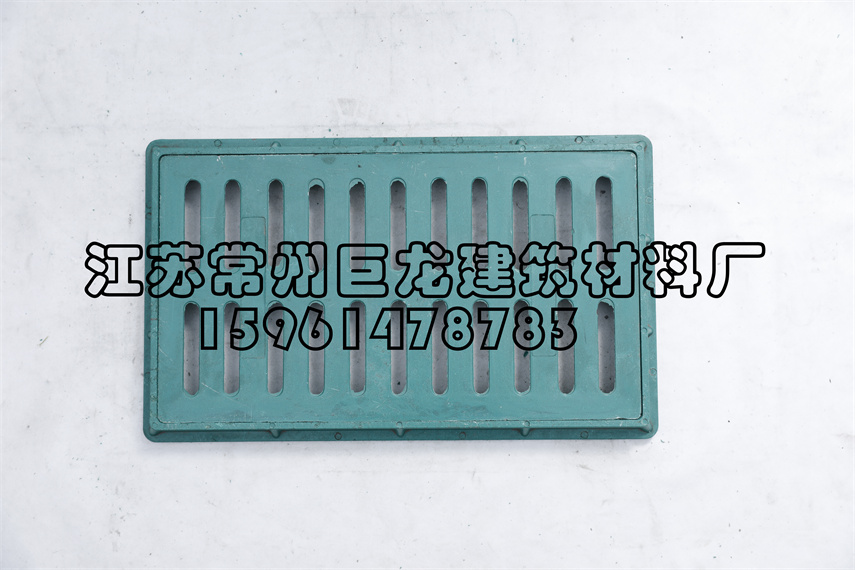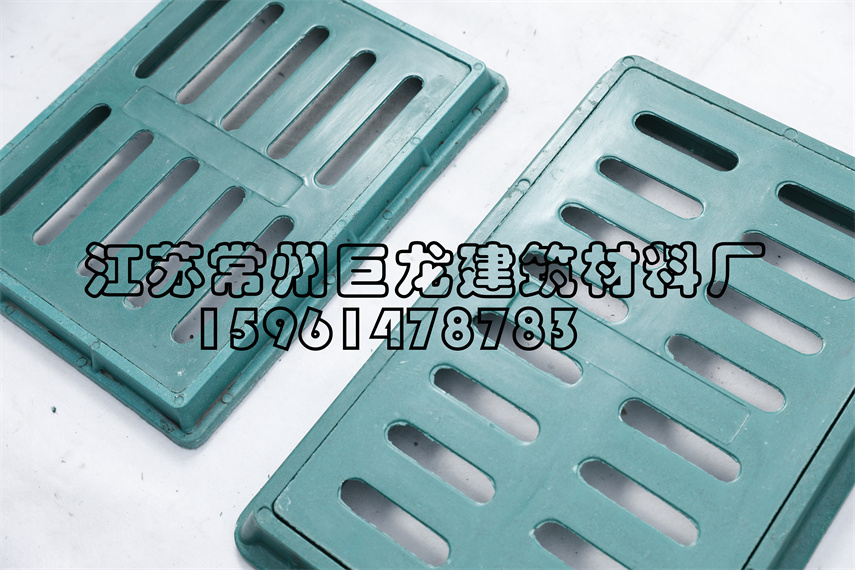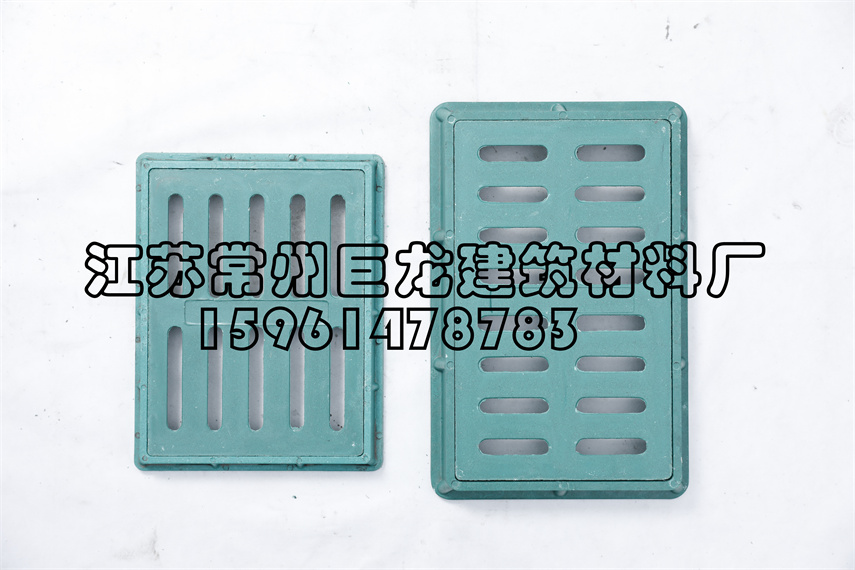### 树脂复合水篦子

#### 作者：江苏常州巨龙建筑材料厂 来源：本站 发布日期：2021-07-06 14:38:10 点击次数：2708

350*250*30=22

300*400*30=32

300*450*40=40

500*300*30=38

500*300*40=52

500*400*30=40

500*400*40=58

500*500*40=70

500*500*50=100

600*400*40=70

600*400*50=100

380*680*40=70

380*680*50=120

750*450*40=75

750*450*50=140公司名称： 联系人： 联系电话： 联系邮箱： 联系地址： 备注：
##### 联系我们

 姓名： 电话： 留言：

• 15961478783
•• 点击扫描下载